# NCERT Solutions for Class 8 Maths Exercise 5.1## myCBSEguide App

CBSE, NCERT, JEE Main, NEET-UG, NDA, Exam Papers, Question Bank, NCERT Solutions, Exemplars, Revision Notes, Free Videos, MCQ Tests & more.

NCERT Solutions for Class 8 Maths Exercise 5.1 Class 8 Maths book solutions are available in PDF format for free download. These ncert book chapter wise questions and answers are very helpful for CBSE exam. CBSE recommends NCERT books and most of the questions in CBSE exam are asked from NCERT text books. Class 8 Maths chapter wise NCERT solution for Maths Book all the chapters can be downloaded from our website and myCBSEguide mobile app for free.

NCERT solutions for Class 8 Maths Data Handling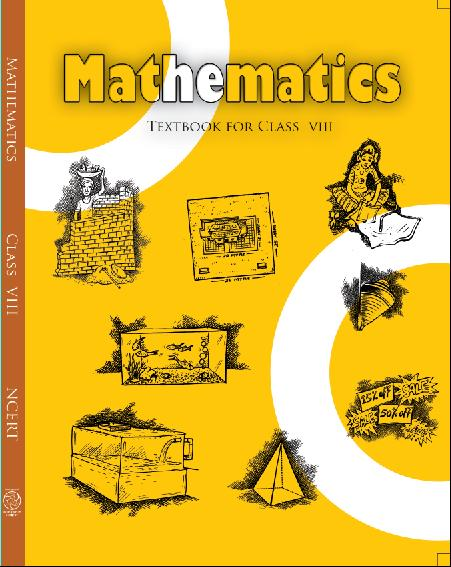## NCERT Solutions for Class 8 Maths Data Handling

###### 1. For which of these would you use a histogram to show the data:

(a) The number of letters for different areas in a postman’s bag.

(b) The height of competitors in an athletics meet.

(c) The number cassettes produced by 5 companies.

(d) The number of passengers boarding trains from 7.00 a.m. to 7.00 p.m. at a station.

Give reason for each.

Ans. Since, Histogram is a graphical representation of data, if data represented in manner of class-interval.

Therefore, for case (b) and (d), we would use a histogram to show the data, because in these cases, data can be divided into class-intervals.

In case (b), a group of competitions having different heights in an athletics meet.

In case (d), the number of passengers boarding trains in an interval of one hour at a station.

NCERT Solutions for Class 8 Maths Exercise 5.1

###### 2. The shoppers who come to a departmental store are marked as: man (M), woman (W), boy (B) or girl (G). The following list gives the shoppers who came during the first hour in the morning.

W WW G B W W M G G M M W WWW G B M W B G G M W W M M W WW M W B W G M W WWW G W M M W M W G W M G W M M B G G W.

Make a frequency distribution table using tally marks. Draw a bar graph to illustrate it.

Ans. The frequency distribution table is as follows: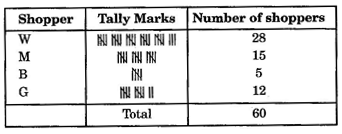The illustration of data by bar-graph is as follows: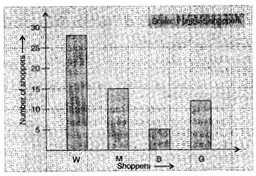NCERT Solutions for Class 8 Maths Exercise 5.1

###### 3. The weekly wages (in ) of 30 workers in a factory are:

830, 835, 890, 810, 835, 836, 869, 845, 898, 890, 820, 860, 832, 833, 855, 845, 804, 808, 812, 840, 885, 835, 835, 836, 878, 840, 868, 890, 806, 840.

Using tally marks, make a frequency table with intervals as 800 – 810, 810 – 820 and so on.

Ans. The representation of data by frequency distribution table using tally marks is as follows: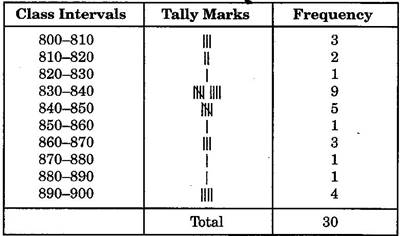NCERT Solutions for Class 8 Maths Exercise 5.1

###### 4. Draw a histogram for the frequency table made for the data in Question 3 and answer the following questions.

(i) How many workers earn  850 and more?

(ii) How many workers earn less than  850?

Ans. 830 – 840 group has the maximum number of workers.

(i) 10 workers can earn more than  850.

(ii) 20 workers earn less than ` 850.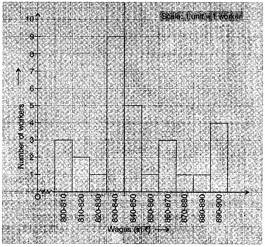NCERT Solutions for Class 8 Maths Exercise 5.1

###### 5. The number of hours for which students of a particular class watched television during holidays is shown through the given graph.

We draw the histogram for above frequency table: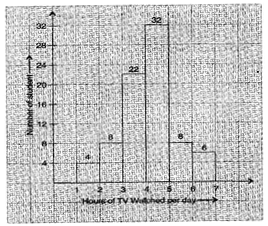(i) For how many hours did the maximum number of students watch T.V.?

(ii) How many students watched TV for less than 4 hours?

(iii) How many students spent more than 5 hours in watching TV?

Ans. (i) The maximum number of students watched T.V. for 4 – 5 hours.

(ii) 34 students watched T.V. for less than 4 hours.

(iii) 14 students spent more than 5 hours in watching T.V.

## NCERT Solutions for Class 8 Maths Exercise 5.1

NCERT Solutions Class 8 Mathematics PDF (Download) Free from myCBSEguide app and myCBSEguide website. Ncert solution class 8 Mathematics includes text book solutions from Class 8 Maths Book . NCERT Solutions for CBSE Class 8 Maths have total 16 chapters. 8 Maths NCERT Solutions in PDF for free Download on our website. Ncert class 8 solutions PDF and Maths ncert class 8 PDF solutions with latest modifications and as per the latest CBSE syllabus are only available in myCBSEguide.

## CBSE app for Class 8

To download NCERT Solutions for class 8 Social Science, Computer Science, Home Science,Hindi ,English, Maths Science do check myCBSEguide app or website. myCBSEguide provides sample papers with solution, test papers for chapter-wise practice, NCERT solutions, NCERT Exemplar solutions, quick revision notes for ready reference, CBSE guess papers and CBSE important question papers. Sample Paper all are made available through the best app for CBSE students and myCBSEguide website.### 1 thought on “NCERT Solutions for Class 8 Maths Exercise 5.1”

1. nice its useful